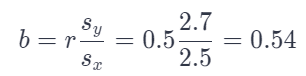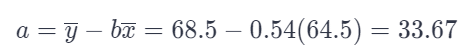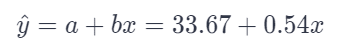# Is the Correlation Between the Heights of Husbands and Wives

We thoroughly check each answer to a question to provide you with the most correct answers. Found a mistake? Let us know about it through the REPORT button at the bottom of the page.

The mean height of married American women in their early twenties is 64.5 inches and the standard deviation is 2.5 inches. The mean height of married men the same age is 68.5 inches, with standard deviation 2.7 inches. The correlation between the heights of husbands and wives is about r = 0.5. Find the equation of the least-squares regression line for predicting a husband’s height from his wife’s height for married couples in their early 20s. Show your work.

Answer: y = 33.67 + 0.54x

## Explanation

r = 0.5

x = 64.5

Sx = 2.5

y = 68.5

Sy = 2.7

General equation of the regression line:

y = a + bx

The slope of the regression line is the linear correlation coefficient multiplied by the standard deviation for y, divided by the standard deviation for x:The intercept is the mean of y decreased by the product of the slope and the mean of x:The equation of the regression line then becomes: